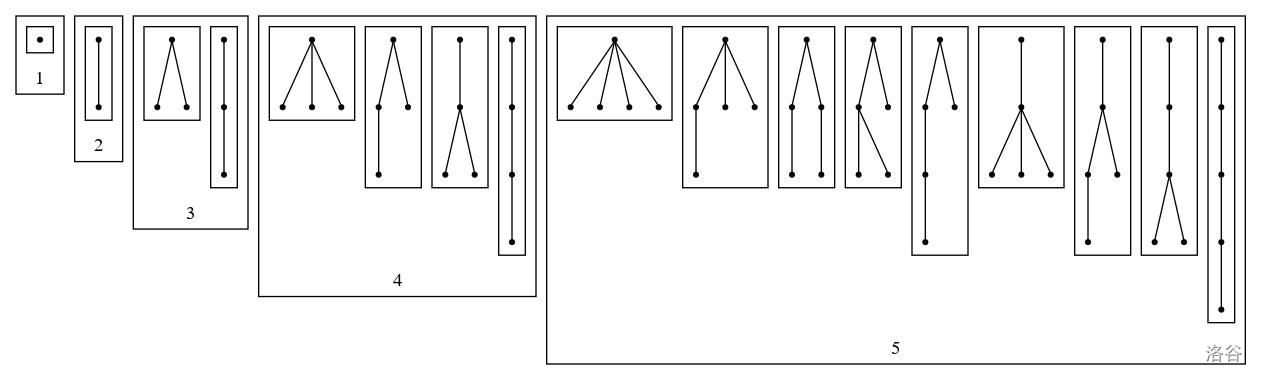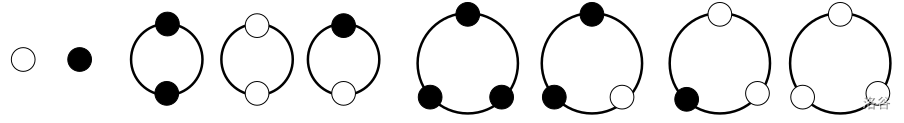2022-07-23 00:40:38

2022年7月30日更新：添加了一些例子和粗体。

# 0. 符号及约定

$x^0=1$ 对所有数成立，包括 $0$。

$\mathbb{N}$ 表示自然数集合，即非负整数集合。

（就好比说 $2$ 的倒数是 $0.5$。但是我们不会说“$0.5$ 是一个倒数”， 只会说“$0.5$ 是一个小数/有理数/分数”。 这里“生成函数”类比“倒数”，“形式幂级数”类比“有理数”）

# 1. 计数类

## 1.1. 计数类

• 对一个元素 $x \in \mathcal{A}$，用 $\lvert x \rvert$ 表示 $x$ 的权值。
• 对于非负整数 $n$，用 $\mathcal{A}_n$ 表示 $\mathcal{A}$ 中所有权值为 $x$ 的元素。
• 用 $A_n$ 表示权值为 $n$ 的元素个数，也就是 $\mathcal{A}_n$ 的大小。我们将非负整数序列 $\{A_0, A_1, \dots\}$ 称为 $\mathcal{A}$ 的计数序列

• 对于非负整数 $n$，$A_n < \infty$。也就是说 $\mathcal{A}$ 中每种权值的元素只有有限个。

• 设 $\mathcal{W}$ 是所有二进制字符串（由 $0$ 和 $1$ 组成的串，包括空串）的集合，其中一个串的权值就是其长度。那么 $\mathcal{W}_n$ 就是所有长为 $n$ 的二进制串的集合，因此 $W_n = 2^n$。
• 设 $\mathcal{F}$ 是上面 $\mathcal{W}$ 的子集，要求二进制串中不能有相邻的两个 $1$。那么 $\mathcal{F}_n$ 就是所有长为 $n$、不包含相邻的 $1$ 的二进制串。我们将会看到，其计数序列 $F_n$ 正是斐波那契数列（习题 2）。
• 设 $\mathcal{B}$ 是无穷大的满二叉树（也就是，从一个根结点出发，每个点都有恰好两个子结点，无穷延伸下去）上所有结点构成的集合，其中每个点的权值是它的深度（根节点深度为 $1$）。不难发现，$B_n = 2^n$，因此 $\mathcal{B} \cong \mathcal{W}$。
• 设 $\mathcal{E} = \{ \epsilon \}$ 是只包含一个权值为 $0$ 的元素的集合（计数类）。因此 $E_0 = 1, E_n = 0 \quad (n \geq 1)$。
• 设 $\mathcal{T}$ 是所有有根树的计数类（这里是无标号的，也就是说两个无根树看作相同当且仅当他们同构），其中每棵树的权值是它的结点个数。则 $T_n$ 就是 $n$ 个点的有根树的个数。我们暂时不知道怎么计算 $T_n$。不过可以列举前几项：$T_0 = 0, T_1 = 1, T_2 = 1, T_3 = 2, T_4 = 4, T_5 = 9$。• 设 $\mathcal{N}$ 是所有“涂了两种色的项链“的计数类：把黑色和白色的两种珠子串成环（可以只用一个珠子），翻转和旋转下一样的项链视作同一个。一条项链的权值就是其中珠子的个数。例如说，不超过四个点的项链共有如下几个。我们列举其计数序列的前几项：$N_1 = 2, N_2 = 3, N_3 = 4, N_4 = 6, N_5 = 8, N_6 = 14, N_7 = 20$。## 1.2 计数构造

（当然，构造也不一定是二元的。它可以是一元的，或者三元的等）。

• 构造得到的类的计数序列只取决于被构造类的计数序列。

• 对两个计数类 $\mathcal{A}, \mathcal{B}$，定义 $\mathcal{A} + \mathcal{B}$ 为这两个类的不交并（即把它们两个里的元素强行视为不相同的，然后取并）。一个元素在这个新计数集里的权值就和在原来的（$\mathcal{A}$ 或者 $\mathcal{B}$）里一样。
• 对两个计数类 $\mathcal{A}, \mathcal{B}$，定义 $\mathcal{A} \times \mathcal{B}$ 为这两个类的乘积。也就是说，这个新类里的元素就是一个两元组（pair）$(a, b)$，其中 $a \in \mathcal{A}, b \in \mathcal{B}$。权值定义为 $\lvert(a, b)\rvert = \lvert a \rvert + \lvert b \rvert$。
• 对一个计数类 $\mathcal{A}$，定义 $\mathtt{SEQ}(\mathcal{A})$ 为以 $\mathcal{A}$ 中元素组成的序列形成的计数类。也就是说 $\mathtt{SEQ}(\mathcal{A})$ 里一个元素就是一个任意长（可以为空）的序列（数组）$(a_1, a_2, \dots, a_n)$，其中每个 $a_i \in \mathcal{A}$。其权值定义为 $\lvert (a_1, a_2, \dots, a_n) \rvert = \lvert a_1 \rvert + \lvert a_2 \rvert + \dots + \lvert a_n \rvert$。
• 对一个计数类 $\mathcal{A}$，定义 $\mathtt{SET}(\mathcal{A})$ 为 $\mathcal{A}$ 的有限子集形成的计数类。也就是说 $\mathtt{SET}(\mathcal{A})$ 里一个元素就是一个子集 $\{a_1, a_2, \dots, a_n\}$，其中每个 $a_i \in \mathcal{A}$。其权值定义为 $\lvert \{a_1, a_2, \dots, a_n\} \rvert = \lvert a_1 \rvert + \lvert a_2 \rvert + \dots + \lvert a_n \rvert$。 这与上一条的区别是，子集中的元素没有顺序，也就是说 $\{ a_1, a_2 \}$ 和 $\{ a_2, a_1 \}$ 视为同一个子集；且子集中每个元素只能出现一次。
• 对一个计数类 $\mathcal{A}$，定义 $\mathtt{MSET}(\mathcal{A})$ 为 $\mathcal{A}$ 的有限多重子集形成的计数类。也就是说 $\mathtt{MSET}(\mathcal{A})$ 里一个元素就是一个多重子集 $\{a_1, a_2, \dots, a_n\}$，其中每个 $a_i \in \mathcal{A}$。其权值定义为 $\lvert \{a_1, a_2, \dots, a_n\} \rvert = \lvert a_1 \rvert + \lvert a_2 \rvert + \dots + \lvert a_n \rvert$。 和上一条基本上相同，除了每个元素可以出现多次。

1. 设 $\{ \bullet \}$ 为一个点构成的构造类，这个点的权值为 $1$。我们把这个构造类本身也记作 $\bullet$。那么：
• $\mathtt{SEQ}(\bullet)$ 中，每种权值的元素恰有一个：权值为 $n$ 的元素就是 $n$ 个 $\bullet$ 组成的序列。我们可以把这个东西看作所有链（图论中的链）的计数类（由于无标号，所以相同点数的链就是同一个）
• $\mathtt{SET}(\bullet)$ 中只有两个元素。一个元素权值为 $0$（空集），另一个元素权值为 $1$（$\{ \bullet \}$）。
• $\mathtt{MSET}(\bullet)$ 和 $\mathtt{SEQ}(\bullet)$ 类似。
2. 设 $\{ \bullet, \circ \}$ 两个点构成的构造类，他们的权值都是 $1$。那么：
• $\mathtt{SEQ}(\{ \bullet, \circ \})$ 中，权值为 $n$ 的元素有 $2^n$ 个。因为权值为 $n$ 的元素就是长为 $n$、每一项或黑或白的序列个数。这个东西显然与二进制串的计数类 $\mathcal{W}$ 等价。
• $\mathtt{SET}(\{ \bullet, \circ \})$ 里共有四个元素，权值分别为 $0, 1, 1, 2$。
• $\mathtt{MSET}(\{ \bullet, \circ \})$ 中权值为 $n$ 的元素有 $n+1$ 个：对每个 $0 \leq i \leq n$，由 $i$ 个黑点和 $n - i$ 个白点构成的多重集的权值都是 $n$。
3. 仍取 1.1 节中的计数类 $\mathcal{W}$。可以把 $\mathcal{W}$ 拆成三部分：空串、以 0 开头的二进制串、以 1 开头的二进制串。第一部分对应计数类 $\{ \epsilon \}$，第二部分对应计数类 $\{ \mathtt{0} \} \times \mathcal{W}$，第三部分对应计数类 $\{ \mathtt{1} \} \times \mathcal{W}$。其中 $\mathtt{0}, \mathtt{1}$ 的权值都为 $1$。因此我们有“组合恒等式”$\mathcal{W} \cong \{ \epsilon \} + (\{ \mathtt{0} \} \times \mathcal{W}) + (\{ \mathtt{1} \} \times \mathcal{W})$。
4. 类似地，我们考虑 1.1 节中的 $\mathcal{F}$。可以把 $\mathcal{F}$ 也拆成三部分：空串、0 开头的串、01 开头的串。因此类似的我们有$\mathcal{F} \cong \{ \epsilon \} + (\{ \mathtt{0} \} \times \mathcal{F}) + (\{ \mathtt{01} \} \times \mathcal{F})$。

## 1.4 习题

1. 给出 1.1 节中，$\mathcal{W}$ 和 $\mathcal{B}$ 之间的一一对应，使得每个元素的权值和它对应的元素的权值相等。
2. 利用 1.2 节中关于 $\mathcal{F}$ 的“组合恒等式”，证明其计数序列 $\{ F_n \}$ 确实是斐波那契数列。
3. 结合 1.2 节中关于 $\mathcal{F}$ 的组合恒等式以及定理 1 的内容，证明其生成函数是 $\frac{1}{1 - x - x^2}$。
4. 用与最后一个“例”或者习题 3 相同的方法，用另一种方式证明 $\mathtt{SEQ}$ 对应的生成函数.（提示：$\mathtt{SEQ}(\mathcal{A}) = \{ \epsilon \} + \mathcal{A} \times \mathtt{SEQ}(\mathcal{A})$）
5. (*) 定理 1 证明中，关于 $\mathtt{SEQ}$ 的部分，我们将无穷多个计数类进行了相加。为什么可以这么做？（提示：有限性）
6. 取 1.1 节中的计数类 $\mathcal{T}$，说明 $\mathcal{T} = \{ \bullet \} \times \mathtt{MSET}(\mathcal{T})$。$\bullet$ 是一个权值为 $1$ 的元素，可以看作树的一个结点。（提示：考虑子树）
7. (*) 证明定理 1 中 $\mathtt{SET}$ 和 $\mathtt{MSET}$ 的等式的后一部分（提示：考虑 $\ln$ 和 $\exp$ 的互逆性质）：$$\prod_{n=1}^\infty (1+x^n)^{A_n} = \exp\Bigl(\sum_{k = 1}^\infty \frac{(-1)^{k - 1}}{k} A(x^k)\Bigr), \quad \prod_{n=1}^\infty \frac{1}{(1-x^n)^{A_n}} = \exp\Bigl(\sum_{k = 1}^\infty \frac{1}{k} A(x^k)\Bigr).$$
8. (**) 给出关于 $\mathtt{SET}$ 和 $\mathtt{MSET}$ 的生成函数的等式的证明。（提示： 01 背包和无穷背包）

• star
首页